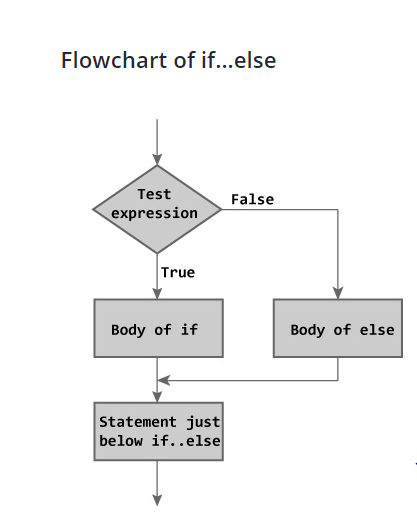×

Search anything:

# if-else control statement

#### control statement if elseGet this book -> Problems on Array: For Interviews and Competitive Programming

if else is a programming construct/ concept for controlling the flow of program execution. The basic idea is to execute different code depending upon the outcome of an expression.

There is three types of programming contructs that fall under this category:

• if
• if else
• if else if

### if

if statement consists of a boolean expression followed by one or more statements which are used to perform different actions based on different conditions.

Syntax of if in C++/ Java:

``````
if (boolean expression)
{
// statements
}
``````

The if statement evaluates the boolean expression inside parenthesis.

If boolean expression is evaluated to true, statements inside the body of if is executed.

If boolean expression is evaluated to false, statements inside the body of if is skipped.

### if else

Now we can easily understand if-else with help of if statement. So let us begin...

An if statement is followed by an optional else statement, which executes when the Boolean expression is false.

Syntax of if else in C++/ Java:

``````
if(boolean expression)
{
// statement(s) will execute if the boolean_expression is true
}
else
{
// statement(s) will execute if the boolean_expression is false
}
``````

The 'if-else' executes the codes inside the body of if statement if the boolean_expression is true and skips the codes inside the body of else.

If the boolean_expression is false, it executes the codes inside the body of else statement and skips the codes inside the body of if.### if else if

if else if can be seen as an extension of if else. In if and if else, only one boolean expression is evaluated but in case of if else if, we can evaluate multiple boolean statements and have finer control.

Syntax in C++/ Java:

``````
if(boolean expression 1)
{
// statement(s) will execute if the boolean_expression_1 is true and previous expressions are false
}
else if(boolean expression 2)
{
// statement(s) will execute if the boolean_expression_2 is true and previous expressions are false
}
else if (another boolean expression)
{
// statement(s) will execute if the boolean_expression_3 is true and previous expressions are false
}
else
{
// statement(s) will execute if all boolean_expressions are false
}
``````

### Example

In this program user is asked to enter a number.we will find whether number is positive or negative with help of if-else statement.
NOTE:'0' is considered as positive.

``````
int main()
{
int number;
cout << "Enter an integer: ";
cin >> number;
if ( number > 0)
{
cout << "You entered a positive integer: " << number << endl;
}
else if (number == 0)
{
cout << "You entered zero: " << number << endl;
}
else
{
cout << "You entered a negative integer: " << number << endl;
}
return 0;
}
``````#### Tanya Anand

Final Year Undergrad (2020) | CSE | NIT Hamirpur | Intern at OpenGenus

if-else control statement